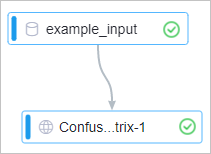The Confusion Matrix component is suitable for supervised learning and corresponds to the matching matrix in unsupervised learning. In precision evaluation, the Confusion Matrix component is used to compare classification results with actual measured values and display the precision of classification results in a matrix.

## Configure the component

You can configure the component by using one of the following methods:
• Use the Machine Learning Platform for AI console
Parameter Description
Original Label Column The columns of numeric data types are supported.
Prediction Result Label Column This parameter is required if the Threshold parameter is not specified.
Threshold The threshold used to determine positive samples. Samples whose sample values are greater than the value of this parameter are positive samples.
Prediction Result Detail Column You can specify only one of the Prediction Result Detail Column and Prediction Result Label Column parameters. This parameter is required if the Threshold parameter is specified.
Positive Sample Label This parameter is required if the Threshold parameter is specified.
• Use commands
• Threshold not specified
``````pai -name confusionmatrix -project algo_public
-DinputTableName=wpbc_pred
-DoutputTableName=wpbc_confu
-DlabelColName=label
-DpredictionColName=prediction_result;``````
• Threshold specified
``````pai -name confusionmatrix -project algo_public
-DinputTableName=wpbc_pred
-DoutputTableName=wpbc_confu
-DlabelColName=label
-DpredictionDetailColName=prediction_detail
-Dthreshold=0.8
-DgoodValue=N;``````
Parameter Required Description Default value
inputTableName Yes The name of the input table. The value is also the name of the prediction output table. N/A
inputTablePartition No The partitions that are selected from the input table for training. Full table
outputTableName Yes The name of the output table. The output table is used to store the confusion matrix. N/A
labelColName Yes The name of the original label column. N/A
predictionColName No The name of the prediction result column. This parameter is required if the threshold parameter is not specified. N/A
predictionDetailColName No The name of the prediction result detail column. This parameter is required if the threshold parameter is specified. N/A
threshold No The threshold used to determine positive samples. 0.5
goodValue No The label value that corresponds to the training coefficient in binary classification. This parameter is required if the threshold parameter is specified. N/A
coreNum No The number of cores used in computing. Determined by the system
memSizePerCore No The memory size of each core. Unit: MB. Determined by the system
lifecycle No The lifecycle of the output table. N/A

## Example

1. Use the following test data as the input.
id label prediction_result
0 A A
1 A B
2 A A
3 A A
4 B B
5 B B
6 B A
7 B B
8 B A
9 A A
2. Create the experiment shown in the following figure. For more information, see Generate a model by using an algorithm.3. Configure the parameters listed in the following table for Confusion Matrix. Retain the default values of the parameters that are not listed in the table.
Parameter Description
Original Label Column Select the label column.
Prediction Result Label Column Set the parameter to prediction_result.
4. Run the experiment and view the output of the Confusion Matrix component.
• Click the Confusion Matrix tab to view the output confusion matrix.
• Click the Proportion Matrix tab to view the proportion matrix.
• Click the Statistics tab to view the statistics of the model.